# Arccos(x) function

Arccos(x), cos-1(x), inverse cosine function.

## Arccos definition

The arccosine of x is defined as the inverse cosine function of x when -1≤x≤1.

When the cosine of y is equal to x:

cos y = x

Then the arccosine of x is equal to the inverse cosine function of x, which is equal to y:

arccos x = cos-1 x = y

(Here cos-1 x means the inverse cosine and does not mean cosine to the power of -1).

#### Example

arccos 1 = cos-1 1 = 0 rad = 0°

## Graph of arccos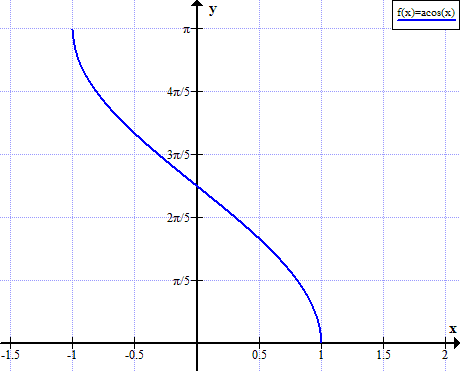## Arccos rules

Rule name Rule
Cosine of arccosine cos( arccos x ) = x
Arccosine of cosine arccos( cos x ) = x + 2kπ, when k∈ℤ (k is integer)
Arccos of negative argument arccos(-x) = π - arccos x = 180° - arccos x
Complementary angles arccos x = π/2 - arcsin x = 90° - arcsin x
Arccos sum arccos(α) + arccos(β) =
arccos( αβ - (1-α2)(1-β2) )
Arccos difference arccos(α) - arccos(β) =
arccos( αβ + (1-α2)(1-β2) )
Arccos of sin of x arccos( sin x ) =  -x - (2k+0.5)π
Sine of arccosine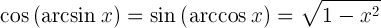Tangent of arccosine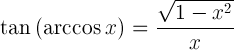Derivative of arccosine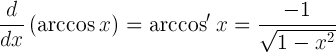Indefinite integral of arccosine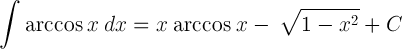## Arccos table

x arccos(x)

arccos(x)

(°)

-1 π 180°
-√3/2 5π/6 150°
-√2/2 3π/4 135°
-1/2 2π/3 120°
0 π/2 90°
1/2 π/3 60°
2/2 π/4 45°
3/2 π/6 30°
1 0

Currently, we have around 1975 calculators, conversion tables and usefull online tools and software features for students, teaching and teachers, designers and simply for everyone.